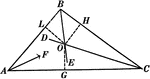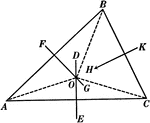Centers of Equilateral Triangle

Illustration of an equilateral triangle that shows both the centroid (where the medians of the sides…Angle Bisectors In A Triangle

Illustration used to prove "The bisectors of the angles of a triangle are concurrent in a point which…Perpendicular Bisectors In A Triangle

Illustration used to prove "The perpendicular bisectors of the sides of a triangle are concurrent in…